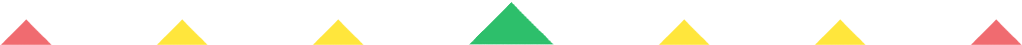Player 1
vs
Player 2
0:00
Opponent history
x
xCorrect
0
Wrong
0
Correct!
Wrong!
Help Sheet for

• 1 = I
• 2 = II
• 3 = III
• 4 = IV
• 5 = V
• 6 = VI
• 7 = VII
• 8 = VIII
• 9 = IX
• 10 = X

• 11 = XI
• 12 = XII
• 13 = XIII
• 14 = XIV
• 15 = XV
• 16 = XVI
• 17 = XVII
• 18 = XVIII
• 19 = XIX
• 20 = XX